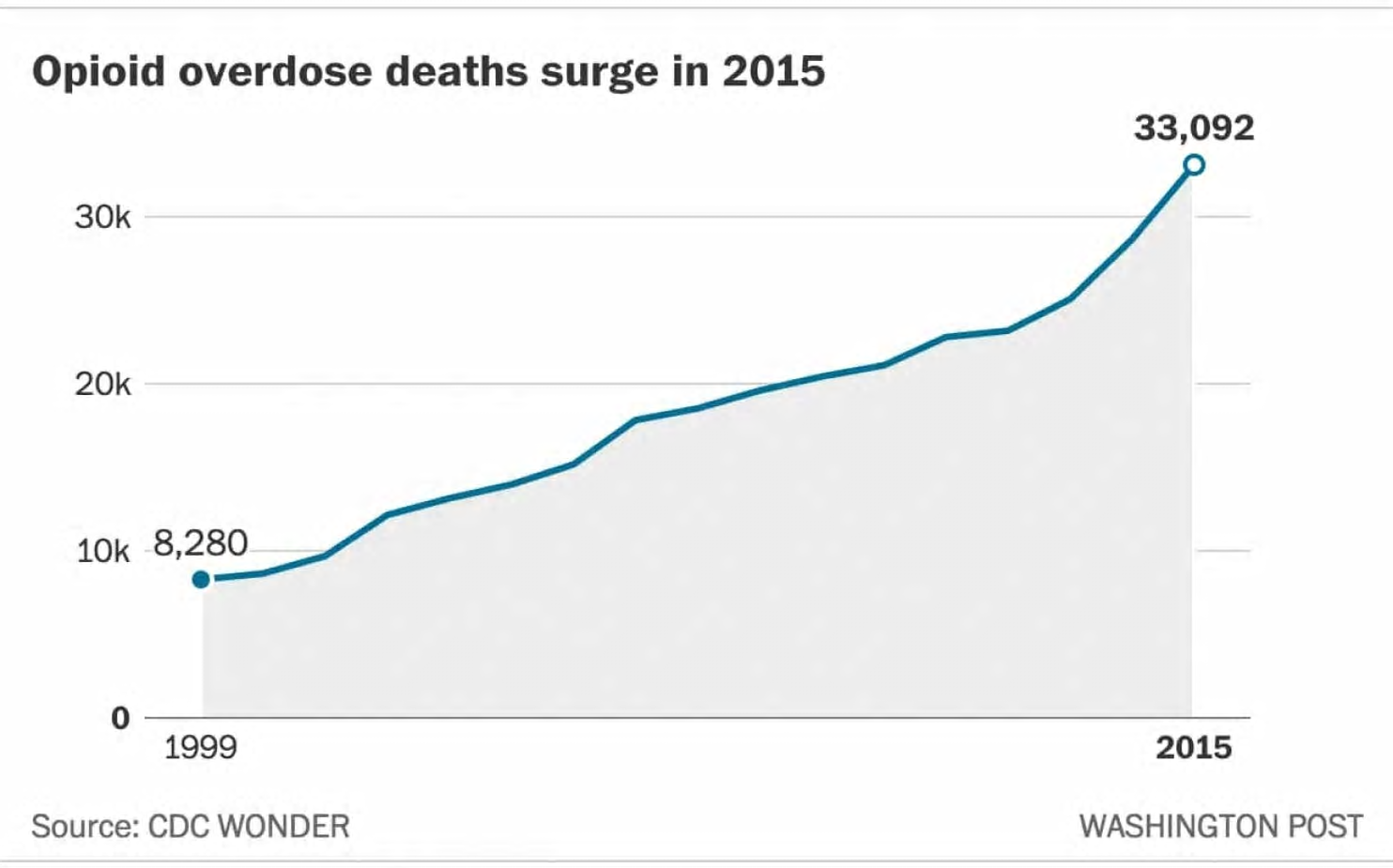Opioid overdose deaths surge in 201533,09230k20k10k 8,280019992015Source: CDC WONDERWASHINGTON POST

Question
• Using 1999 as year zero (0), and 2015,
• A. Construct an exponential equation that models the “Opioid overdose deaths surge in 2015” chart.
•
• From your model state the average yearly percent change from 1999 to 2015.
• Use your model to extrapolate the number of deaths in 2020.help_outlineImage TranscriptioncloseOpioid overdose deaths surge in 2015 33,092 30k 20k 10k 8,280 0 1999 2015 Source: CDC WONDER WASHINGTON POST fullscreen
Step 1

Explanation: Part A:

Denote x as the year, which is the independent variable and y as the number of deaths due to opioid overdose.

If 1999 is year 0, then the first available value of x is, x1 = 0. Corresponding value of y is, y1 = 8,280.

Now, 2015 – 1999 = 16. So, if value of x is 0 for 1999, value of x will be 16 for 2015.

Thus, the second available value of x is, x2 = 16. Corresponding value of y is, y2 = 33,092.

Now, an exponential model is of the form: y = abx. Using the values above, a and b can be found as follows, thus creating the model:

Step 2

Average yearly percent change:

In the exponential model of the above form: y = abx, “b” is commonly referred to as the “growth factor”. If b < 1, then there is a reduction or decay over time, whereas if b > 1, then there is a growth or increase over time.

For b > 1, that is, in case of growth, b can be expressed as, b = 1 + r, where r is the percentage or proportion of growth, attained over each unit increase in time.

Here, b = 1.09, that is, 1 + r = 1.09; r = 0.09 = 9% (= 0.09 × 100%).

Thus, the average yearly percent change from 1999 to 2015 is approximately 9%.

Step 3

Extrapolation for 2020:

The year 2020 is 5 years from 2015, which is the year 16. So, for 2020, x = 21 (= 16 + 5). Substitute thi...

Want to see the full answer?

See Solution

Want to see this answer and more?

Our solutions are written by experts, many with advanced degrees, and available 24/7

See Solution
Tagged in

Other